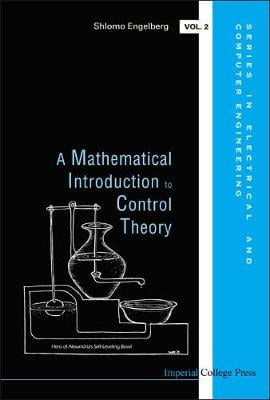•Mathematical Introduction To Control Theory, A - Series in Electrical and Computer Engineering 2 (Hardback)

(author)
£100.00
Hardback 368 Pages / Published: 02/06/2005
• We can order this

Usually dispatched within 3 weeks

Striking a careful balance between mathematical rigor and engineering-oriented applications, this textbook aims to maximize the readers' understanding of both the mathematical and engineering aspects of control theory.The bedrock elements of classical control theory are comprehensively covered: the Routh-Hurwitz theorem and applications, Nyquist diagrams, Bode plots, root locus plots, the design of controllers (phase-lag, phase-lead, lag-lead, and PID), and three further advanced topics: non-linear control, modern control and discrete-time control.A Mathematical Introduction to Control Theory will be an invaluable book for junior and senior level university students in engineering, particularly electrical engineering. Students with a good knowledge of algebra and complex variables will also find many interesting applications in this volume.

Publisher: Imperial College Press
ISBN: 9781860945700
Number of pages: 368
Weight: 635 g
Dimensions: 232 x 159 x 27 mm